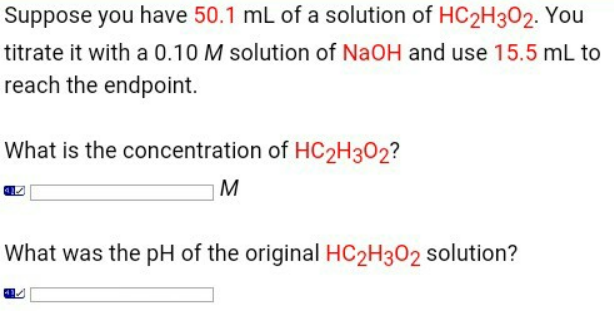# Problem: Suppose you have 50.1 mL of a solution of HC 2H3O2. You titrate it with a 0.10 M solution of NaOH and use 15.5 mL to reach the endpoint. What is the concentration of HC2H3O2?What was the pH of the original HC 2H3O2 solution?

###### FREE Expert Solution
94% (126 ratings)###### Problem Details

Suppose you have 50.1 mL of a solution of HC 2H3O2. You titrate it with a 0.10 M solution of NaOH and use 15.5 mL to reach the endpoint.

What is the concentration of HC2H3O2?

What was the pH of the original HC 2H3O2 solution?